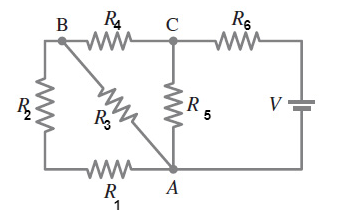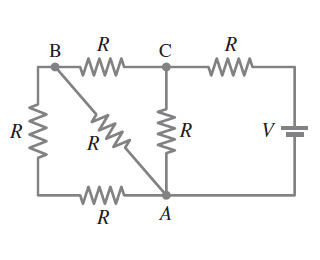# Problem: What is the net resistance of the circuit connected to the battery in (Figure 1)?Express your answer in terms of R.

###### FREE Expert Solution

Equivalent resistance for resistors in series:

$\overline{){{\mathbf{R}}}_{\mathbf{e}\mathbf{q}}{\mathbf{=}}{{\mathbf{R}}}_{{\mathbf{1}}}{\mathbf{+}}{{\mathbf{R}}}_{{\mathbf{2}}}{\mathbf{+}}{\mathbf{.}}{\mathbf{.}}{\mathbf{.}}{\mathbf{+}}{{\mathbf{R}}}_{{\mathbf{n}}}}$

Equivalent resistor for resistors in parallel:

$\overline{)\frac{\mathbf{1}}{{\mathbf{R}}_{\mathbf{e}\mathbf{q}}}{\mathbf{=}}\frac{\mathbf{1}}{{\mathbf{R}}_{\mathbf{1}}}{\mathbf{+}}\frac{\mathbf{1}}{{\mathbf{R}}_{\mathbf{2}}}{\mathbf{+}}{\mathbf{.}}{\mathbf{.}}{\mathbf{.}}{\mathbf{+}}\frac{\mathbf{1}}{{\mathbf{R}}_{\mathbf{n}}}}$

Let's label the resistors as follows:Resistors 1 and 2 are in series.

R12 = R1 + R2 = R + R = 2R

R12 and R3 are in parallel.

1/R123 = 1/2R + 1/R = 3/2R

99% (152 ratings)###### Problem Details

What is the net resistance of the circuit connected to the battery in (Figure 1)?

Express your answer in terms of R.Frequently Asked Questions

What scientific concept do you need to know in order to solve this problem?

Our tutors have indicated that to solve this problem you will need to apply the Combining Resistors in Series & Parallel concept. You can view video lessons to learn Combining Resistors in Series & Parallel. Or if you need more Combining Resistors in Series & Parallel practice, you can also practice Combining Resistors in Series & Parallel practice problems.

What professor is this problem relevant for?

Based on our data, we think this problem is relevant for Professor Memari's class at NSU.